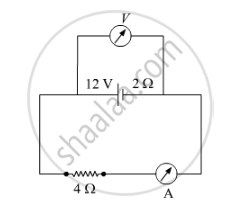Share

# A Battery of Emf 12 V and Internal Resistance 2 Ω is Connected to a 4 Ω Resistor as Shown in the Figure. (A) Show that a Voltmeter When Placed Across the Cell and Across the Resistor, in Turn, Gives the Same Reading. - CBSE (Science) Class 12 - Physics

#### Question

A battery of emf 12 V and internal resistance 2 Ω is connected to a 4 Ω resistor as shown in the figure.

(a) Show that a voltmeter when placed across the cell and across the resistor, in turn, gives the same reading.

(b) To record the voltage and the current in the circuit, why is voltmeter placed in parallel and ammeter in series in the circuit?#### Solution

(i) According to the definition of terminal potential difference,

E = V + Ir

=>V=E-Ir

E = 12 V, r = 2 Ω

V = 12 – 2I

When the voltmeter is connected across the cell.

I=12/(4+2)=2A

V1 = 12 – 2 (2) = 8 V

When the voltmeter is connected across the resistor.

V2 = IR

= 2 × 4 = 8 V

V1 = V2

Hence, from the above relation we can see that when voltmeter placed across the cell and across the resistor, gives the same reading

(ii) Voltmeter has very high resistance to ensure that it's connection does not alter the flow of current in the circuit. We connect it in parallel and we also know that current chooses only the low resistance path. Hence, it is connected in parallel to the load across which potential difference is to be measured.

Ammeter measures value of current flowing through the circuit so it should be connected in the series. Ammeter has very low resistance to ensure that all the current flows through it. Thus, it gives a correct reading of the current when connected in series.

Is there an error in this question or solution?

#### Video TutorialsVIEW ALL 

Solution A Battery of Emf 12 V and Internal Resistance 2 Ω is Connected to a 4 Ω Resistor as Shown in the Figure. (A) Show that a Voltmeter When Placed Across the Cell and Across the Resistor, in Turn, Gives the Same Reading. Concept: Cells, Emf, Internal Resistance.
S